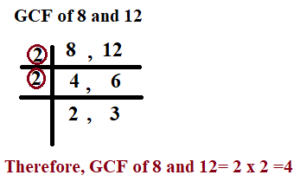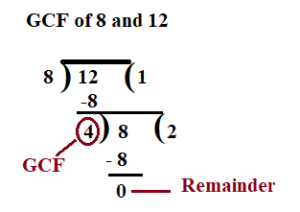October 7, 2022

# Greatest Common Factor of 8 and 12 | Methods to find GCF of 8 and 12

Greatest Common Factor of 8 and 12

# Find the  Greatest Common Factor of 8 and 12.

Methods to Find GCF of 8 and 12

1. Factorisation Method
2. Prime Factorisation Method
3. Common Division Method
4.  Division Method

## 1. Greatest Common Factor of 8 and 12 by Factorisation Method

We have  8= 1 x 8 ;    8=2 x 4

All the factors of 8 are  :  1, 2, 4, 8

Again  12= 1 x 12 ;   12 =2 x 6  ; 12= 3 x 4

So , all the factors of 12 are :  1 , 2, 3, 4, 6, 12

Common factors of 8 and 12 are : 1, 2, 4

Hence, the  Greatest Common Factor of 8 and 12 or HCF of   8 and 12  is  4.

### 2. Greatest Common Factor of 8 and 12 by  Prime Factorisation Method

By prime factorisation, we have :

8= 2 x 2 x 2

12= 2 x 2 x 3

Greatest Common Factor of 8 and 12 = product of common prime factors of 8 and 12

= 2 x 2 =4

Hence , the GCF of 8 and 12 is 4.

#### 3. Greatest Common Factor of 8 and 12 by Common Division Method

Common Division Method : The steps for Common Division Method are given below:

Step 1:  Arrange  the given numbers in a line in any order.

Step 2: Divide the numbers by the lowest common prime number. Write the quotients below the numbers.

Step 3: Then divide the quotients by the lowest common prime number.

Step 4: Repeat the process till there are no common prime numbers to divide by.

Step 5 : The product of the divisors is the required GCF.

Greatest Common Factor of 8 and 12 by common division method

We have##### 4. GCF of 8 and 12 by Division MethodSo GCF of 8 and 12 is = 4

You might also be interested in:#### Bina singh

View all posts by Bina singh →
List of Google Scholarships IBPS Clerk 2022 Mains Exam Admit card 2022 CUET-PG results Scheduled Exams in October 2022 SBI Notification for the 1673 Probationary Officers